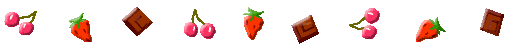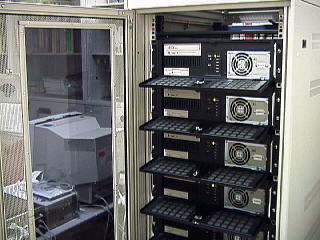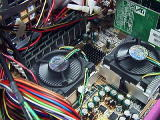Materials Science Study by Molecular Dynamics

1. Method

When we study high-temperature plasmas and the microscopic
structure and functionality of condensed matters by computer
simulations, we use either classical molecular dynamics code
based on Newtonian dynamics, or the first principle code based
on quantum mechanics, depending on the scale and the process
to be studied. The former code can deal with the stellar motions
in the universe down to atomic motions in nanometer scales.
The latter code should be used for the study that includes atomic
processes where overlapping of atoms, hence that of electron
orbits, takes place.

The electrostatic forces are inverse-square forces like the
gravitational forces, which are long-range forces in nature. But,
one has to calculate them correctly also for short distances since
particle near-by collisions are frequent. Thus, we take summation
of the short-range part of the electrostatic forces over all the ion
combinations. This requires N*N calculations on each time step
of the simulation run, where N is the number of ions in the system.
If we integrate the equations for, at least, 100,000 steps and with
N= 1000, the total of 100 billion calculations are executed in the
run. Thus, we must use a fast computer for molecular dynamics
simulations.

In the classical molecular dynamics, the most time consuming
part of computation is the Ewald sum which takes into account
the periodicity of the charge distribution（typical in crystals).
However, the equations are rather simple. By contrast, the
first-principle molecular dynamics code solves the equation for
electron density, which requires large matrix diagonalization.
These procedures are rather complicated and even heavier in
computation than solving equations of motion.

2. PC Cluster MachinesAs explained above, molecular dynamics
simulations need very fast computers, and
only supercomputers could do the job
before. Now, the x86-based PC cluster
machines are replacing conventional
supercomputers bacuase of their capability
of handling large amount of computations.
Thus, if one can wisely parallelize the
molecular dynamics code, a Boewulf PC
cluster (parallel computer) is highly suited for
molecular dynamics simulations.For these reasons, we constructed several
clusters based on Pentium III (1GHz), Xeon
(2.4GHz), Pentium 4 (3GHz) with MPICH.
We found later that using the low-latency
communication software GAMMA doubles
the computation power.
Recently we use Opteron 2.8 GHz clusters
with the SuSE Linux ES and the InfiniBand
MPI for inter-processor communications.
We are making good use of these cluster
machines in our current research of plasma
and condensed matters.

Topics

Boewulf PC cluster: How to make and use it (summary)

Our Boewulf PC Cluster Machine　(report)

Xeon Processors and Remarks May 2003

Performance of Pentium 4 Processors Oct. 2003Home